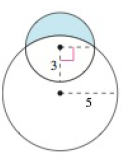Chapter 8.4, Problem 67E

Chapter
Section
Textbook Problem

Area of a LuneThe crescent shaped region bounded by two circles forms a lune (see figure).Find the area of the lune given that the radius of the smaller circle is 3 and the radius of the larger circle is 5.To determine

To calculate: The area of the lune.

Explanation

Given: Two circles, such that the radius of the smaller circle is 3 and the radius of the larger circle is 5.

Refer to the question for figure.

Calculation:

A=33(4+32x252x2)dxBecause of the symmetry in the y axis, you can rewrite the integral as:A=203(4+32x252x2)dxUse the Integration formula,a2u2du=12[u<

Still sussing out bartleby?

Check out a sample textbook solution.

See a sample solution

The Solution to Your Study Problems

Bartleby provides explanations to thousands of textbook problems written by our experts, many with advanced degrees!

Get Started

If a and b are positive numbers, show that 01xa(1x)bdx=01xb(1x)adx

Single Variable Calculus: Early Transcendentals, Volume I

In problems 63-73, factor each expression completely. 69.

Mathematical Applications for the Management, Life, and Social Sciences

Sometimes, Always, or Never: fxy = fyx.

Study Guide for Stewart's Multivariable Calculus, 8th

Identify the basic goals for descriptive statistics and for inferential statistics.

Research Methods for the Behavioral Sciences (MindTap Course List)